## Light Fields

CS 348B - Computer Graphics: Image Synthesis Techniques
Spring Quarter, 1997
(TA) Lucas Pereira
Lecture notes for Tuesday, May 13, 1997
Handout #26

## Light Fields

### Contents:

• Introduction: Image-based rendering
• Background
• The light field
• Light field parameterization
• Is this really rendering at all?
• Sampling issues
• The line space dual
• Light field generation
• Light field compression

### Introduction: Image-based rendering

• Image-based rendering - Creating new views of a 3D environment based on existing images
• Why would we want image-based rendering?
• Speed, Realism
• Raytracers can't do 30 frames a second
• Infinite Not-Quite-Reality
• Speed, independent of scene complexity
• Source of images: real or synthetic
• Memory usage
• Finite Resolution
• Possibly high precomputation costs (such as raytracing a billion pixels)
• Where have we seen image-based approaches before?
• Texture-mapping
• Faster than a million polygons
• More realistic than a Gouraud-interpolated plane
• Environment-mapping
• Direction-dependent
• "Environment" picture mapped onto planes that don't really exist, but provide a nice parameterization

### Background

• Chen & Williams (Siggraph '93)
• When doing real-time fly-throughs, images are coherent (similar)
• Morphing in-between images faster than rendering every image
• Computing the warp: depends on object depth
• Inverse velocity gives depth
• Differences in depth cause missing pixels
• QuickTime VR
• panorama: 360-degree field of view, discrete locations
• Viewer "jumps" from one discrete location to another
• object: discrete locations around the object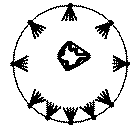### The light field

• Samples the 4D flow of light in free space.
• Can reconstruct any view inside the convex hull (for outward-looking) or outside the convex hull (for inward-looking)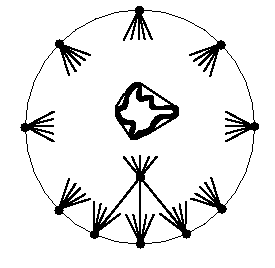• Webster Definitions:
• Light - electromagnetic energy in the visible spectrum
• Field - a region or space in which a given effect (such as magnetism) exists
• Render - to reproduce or represent
• Light field Rendering - To reproduce or represent an object using the distribution of visible electromagnetic energy over a region of space
• Plenoptic Field - from latin "plenum", which was once thought to be the ether through which light flowed

### Light Field Parameterization

• Fully general parameterization: from every point in space x,y,z (3D), x the light travelling in every direction s,t (2D), = 5D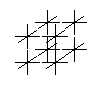• Equivalent to having an environment map for every point in space
• Expensive - in free space, rays don't change along their length
• 2-Plane parameterization: light in free space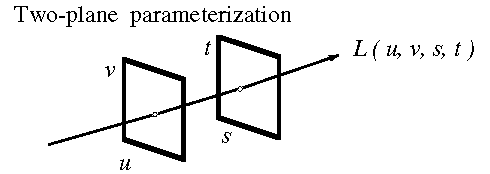• Each u,v,s,t specifies a unique line in freespace
• Planes can be arbitrarily placed, even at infinity
• Singularities: intersections, both planes at infinity
• Everything is linear - an image from anywhere in space is a planar 2D "slice" in the 4D volume
• This makes it easy for computers to compute quickly

### Is this really rendering at all?

• Rendering: Generation of a 2D image from a 3D scene (from lecture #1)
• Light fields satisfy that definition
• Ways to think about light fields
1. A rendering method
2. An object representation format
3. A caching method
4. A hidden-surface algorithm, with reflectance maps
5. An object simplification algorithm

### Sampling issues

• Prefilter: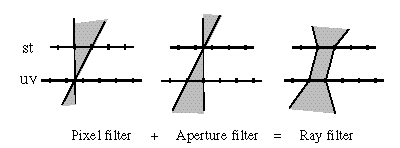• Each u,v,s,t pixel represents all the rays that pass through these two filter regions
• Postfilter:
• Quadralinear interpolation: convolve triangle filter in all 4 dimensions
• This can be implemented as a bilinear interpolation between 4 bilinearly interpolated samples
• 16 samples contribute to each screen pixel
• Faster: bilinear interpolation
• Choose only two dimensions to filter, such as u-v
• If we do this, we might want more resolution in the un-filtered two dimensions
• Example: typical light field 64x64 in uv, 256x256 in st
• Fastest: point sampling
• Grab the closest sample
• See "jaggies" both in uv, and st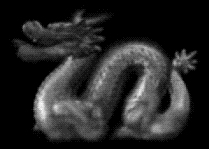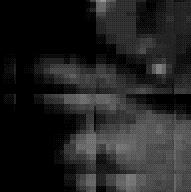• Minification:
• Can build multiresolution pyramid, which would be 5D (An linear array of 4D structures)
• Each level up is 1/16 the size -- So cost of the pyramid is trivial

### The line space dual

• Nonuniform spatial resolution
• A light field does not have uniform resolution throughout space
• Uniform resolution impossible:
• Memory is finite
• 4D space is infinite
• Uniform resolution everywhere means average resolution is (memorysize / infinity), which is 0
• Nonuniform resolution hard to visualize
• What do we care about, to generate an image of light field?
• From a given viewpoint, what is the sample spacing
• From a given viewpoint, what is the angular distribution
• The line space dual (in 2D)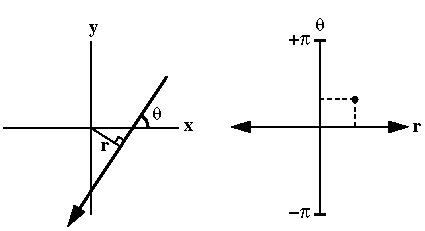• Lines in Cartesian space map to points in line space
• Parallel lines map to a horizontal line in line space
• Lines that pass through a single point map to a vertical line in line space
• Density of points in line space show light field resolution in Cartesian space

### Light field generation

• Object placement
• Typically, a surface has higher spatial variation than angular variation
• Remember bilinear filtering is cheaper, so we often have nonuniform spatial resolution
• Place object so that its spatial variation corresponds to highest region of spatial resolution
• If st plane is higher resolution, place object near st plane
• Often refer to st plane as "focal plane", and uv as "camera plane"
• Effects of low linespace resolution: blurring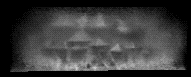• Sheared projection
• Each view from a uv "camera location" is a sheared projection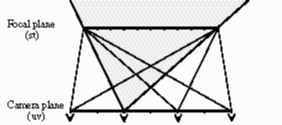• For acquired light fields, camera was rotated instead of sheared, so we have to warp the images
• For synthetic light fields, shear is not a problem

### Light Field Compression

• Two big disadvantages: memory usage, finite resolution
• Closely related: without memory restrictions, we could do far higher resolution
• Solution: compression
• Light fields have huge coherence
• Each closely-spaced image is very similar
• Surface reflection map generally low frequency
• Current solution: vector quantization
• Codebook represents block of pixels
• Codebook learned on "training set"
• Indices choose best codebook for each tile of light field
• Typical:
• 2-byte index (64K Codebook entries)
• 2x2x2x2 pixels, x3 colors = 48 bytes
• compression: 48:2, or 24:1
• Example: Buddha light field
• 32 x 32 x 256 x 256 x 3 = 192 MB
• 24:1 compression = 8 MB of indices
• 32K codebook entries x 48 bytes = 1.5 MB
• Total size: 8 + 1.5 = 9.5 MB
• Future solution: "motion" estimation?
• In epipolar space (e.g. u-s), points in space form lines in a light field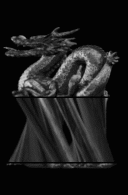• Slope of the line depends on depth of the point
• We are trying to represent this set of lines as a textured polygon and motion vectors
• Similar to mpeg, in theory
• In practice, needs to be random-access for rendering
• Calculating "velocity" of the planes equal to Chen & Williams calculating depth
• Then, just render the textured polygon in the right place, to get 4D sample
• Once again, we only filtering in 2D
• This is reminiscent of hidden surface algorithm, where for each local area, there is only one valid plane
• This is the topic of my current research (along with Ben Zhu)

lucasp@graphics.stanford.edu